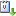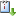##### Personal tools
You are here: Home / 2012 / RISC Colloquium

# RISC Colloquium

Prof. Petr Lisonek: Classical modular polynomials over GF(2)
When Sep 24, 2012 from 01:30 PM to 02:30 PM Seminar RoomvCaliCal

For a prime number l, the classical modular polynomial Phi_l (sometimes called the modular equation of level l) is a polynomial with integer coefficients such that Phi_l(j(t),j(lt))=0 where j(t) is the j-invariant well known from the theory of elliptic functions.  While it is difficult to compute Phi_l over the integers due to the rapid growth of its coefficients, there is a beautiful structure emerging if one considers Phi_l over GF(2), where it becomes a sparse polynomial.  Even though the modular polynomials considered over GF(2) have important computational applications in elliptic curves cryptography, it appears that the amazing structure of their non-vanishing terms has not been considered in the literature.  By considering the power series expansion of j(q), we prove some necessary conditions that a monomial of Phi_l must satisfy in order to have coefficient 1, and we conjecture many more such conditions based on results from our computational investigations.

« August 2020 »
August
MoTuWeThFrSaSu
12
3456789
10111213141516
17181920212223
24252627282930
31